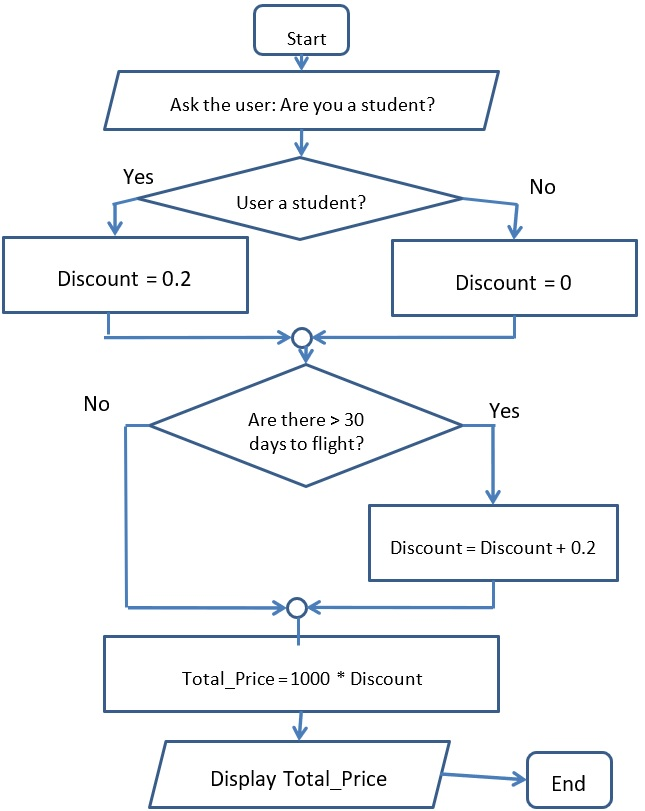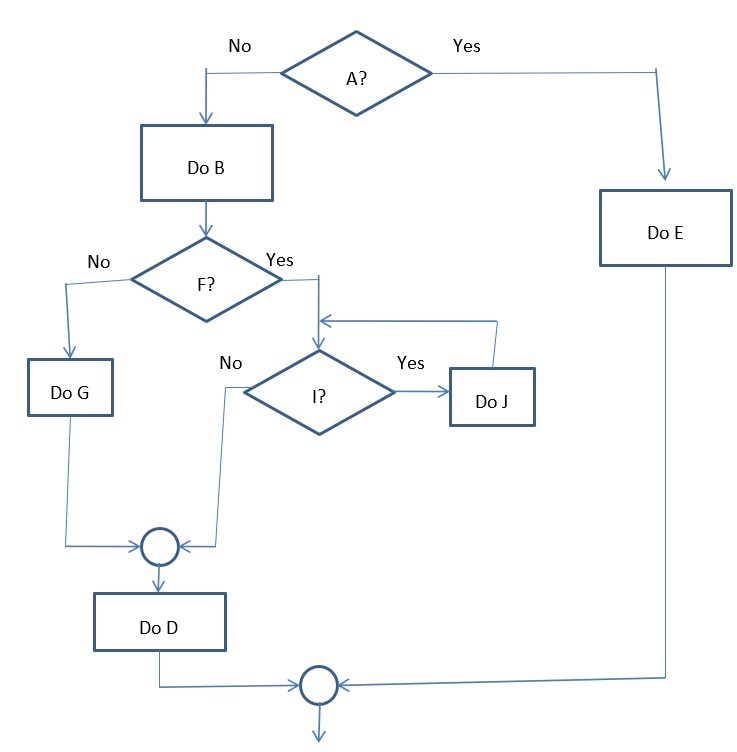# Write a function to return the character

Section 1 [10 point—each 1 point]: Multiple choice questions (Select only ONE answer for each question)

• Visual Basic is considered to be a
1. first-generation language. B) package. C) high-level language. D) machine language.
• Which type of procedure returns a value?
1. Sub Procedure B) Procedure C) Function D) ByVal
• Which of the following is NOT a valid filename in Windows?
1. MYFILE*.TXT B) _MYFILE.TXT C) MYDATA.TXT D) MY-FILE.TXT
• An algorithm is defined as
1. a mathematical formula that solves a problem.
2. a tempo for classical music played in a coda.
3. a logical sequence of a steps that solve a problem.
4. a tool that designs computer programs and draws the user interface.
• Which of the following is the proper order of procedures used in the problem solving process?
1. analysis, design, coding, testing B) analysis, testing, design, coding
2. C) design, analysis, coding, testing D) analysis, design, testing, coding E) design, testing, analysis, coding F) design, coding, testing, analysis
• Which is not a proper looping structure?
1. Do While B) Loop Until C) Do Until D) For … Next
• Which one of the following is NOT one of the three basic types of statement structures?
1. sequence B) loop C) decision D) input/output
• What does the diamond flowchart symbol represent?
1. input/output B) terminal C) decision D) connector E) process
• A graphical depiction of the logical steps to carry out a task and show how the steps relate to each other is called a(n)
1. B) pseudocode. C) algorithms. D) hierarchy chart.
• What would be a good name for a text box to hold a person‟s first name?
1. FirstName B) txtFirstName C) textBoxFirstName D) boxFirstName

tion 2 [10 points]: Multiple-choice questions (Select only ONE answer for each question)

(1) Which of the following is the correct statement for specifying the words to appear in the title bar of Form called Form1?

 [1 point] A) Form1.Text = “My Text” B) Form1.TitleBar = “My Text” C) Me.Text = “My Text” D) Me.Caption = “My Text” (2) What will be the content of the variable x after the following statement is executed? x = Math.Sqrt((9 + 7) / (4 * 2) + 2) A) 1 B) 2 C) 3 D) 4 E) None of the above [1 point] (3) What will be displayed when the following lines are executed? Dim x As Double = 3, y as Double = 1 Dim z As Double z = x + (y * x) x = y z = x + z lstBox.Items.Add(x + y + z) A) 4 B) 9 C) 10 D) None of the above [2 points] (4) What will be displayed when the following lines are executed? [2 points]

Dim a, b, c As String a = “THE WHOLE” b = “PART” c = a.Substring(CInt(Math.Sqrt(4)), b.Length) txtBox.Text = CStr(c)

1. A) HE W B) E WH C) THE W D) THE WHOLE PART
• What is a valid input if a Masked Text Box has the following mask: “(00) 0&LLL” [1 point] A) “(33) 3aaaa” B) “(00) ACDEF” C) “(00) 0&LLL” D) “(83)d9OST”
• What is the result of the following code? [1 point]

Dim Boy as string = “Jack” Dim Girl as string = “Jill”

txtBox.Text = Boy & “ and “ & “Girl” & “ went up the hill”

1. Nothing, there‟s an error. B) “Jack and Jill went up the hill”
2. C) “Jack and Girl went up the hill” D) “ and went up the hill”
• Which one of the following is NOT one of the three main types of errors? [1 point] A) Syntax error B) Logic error C) Declaration error D) Run-time error
• What type of numeric variable type holds the most data? [1 point]
1. Short B) Long C) Integer D) Single E) Double

tion 3 [15 points]:

• Simplify the code. [3 points]

If (a < b) Then If (b < c) Then

txtOutput.Text = b & “ is between “ & a & “ and “ & c

End If

• What will be the output of the following program when the button is clicked? [6 points]

Private Sub btnDisplay_Click(...) Handels btnDisplay.Click Dim word1, word2, newWord As String word1 = “memory” word2 = “feather”

newWord = word1.Substring(2,2) & word2.Substring(3,4) If newWord.Length > 3 Then txtBox.Text = newWord Else

End If End Sub

Output:

(3) What does the following code display? [6 points]

Private Sub btnDisplay_Click(…) Handles btnDisplay.Click Dim amt1 As Integer = 1, amt2 As Integer = 2 lstOutput.Items.Add(amt1 & “ ” & amt2) Swap(amt1, amt2)

lstOutput.Items.Add(amt1 & “ ” & amt2)

End Sub

Sub Swap(ByRef num1 As Integer, ByVal num2 As Integer) Dim temp As Integer temp = num1 num1 = num2 num2 = temp

lstOutput.Items.Add(num1 & “ ” & num2) num2 = 3

End Sub

Output:

tion 4 [20 points]

• The price of a plane ticket is 1000\$ by default, but discounts are applied to it based on different criteria. The following rules determine the discount, and hence the final price:
• Students get 20% discount.
• People who purchase in 30 days in advance get 25% discount.

Discount can aggregate, for example a student purchasing 40 days in advance gets a 40% discount. You have to ask the user for input on whether they are a student. Draw a flowchart of your algorithm that solves

the following problem and calculates the final price. [10 points]• Translate the following flowchart to pseudo code. [10 points]```{`
If A Then
Do E
Else
Do B
If F Then
Do While I is true
Do J
Loop
Else
Do G
End If
Do D
End If
`}```

Section 5 [10 points]:

• Answer the following questions about the value of Z, if [5 points]

Z = (X AND Y) OR (X AND (NOT Y))

1. If X = TRUE and Y = FALSE then Z =
1. If X = TRUE and Y = TRUE then Z =
1. If X = FALSE and Y = FALSE then Z =
1. If X = FALSE and Y = TRUE then Z =
1. Is X = Z?
• What will be the output of the following code when it is executed? [5 points]

Dim age As Integer = 42

Select Case age Case Is >= 0

txtBox.Text = “Child” Case Is >= 13

txtBox.Text = “Teenager” Case Is >= 21

txtBox.Text = “Young Adult” Case Is >= 40

txtBox.Text = “Middle Age” Case Is >= 65

txtBox.Text = “Old Person” Case Else

txtBox.Text = “Not Human”

End Select

Output:

Section 6 [10 points]

(1) Write a function to return the character which follows the input character according to the ASCII table. For example, if the user specifies „A‟, the function has to return „B‟. No error checking necessary. Write a statement which illustrates how to use this function. Please write comments in the code to explain what is going on.

Private Sub Button1_Click(…) Handles Button1.Click

Dim InputString As String

InputString = InputBox({"Enter a character"})

MsgBox(NextChar(InputString))

End Sub

Private Function NextChar(ByVal InputString As String) As String

Dim AsciiCode As Integer

AsciiCode = Asc(InputString)

AsciiCode = AsciiCode + 1

NextChar = Chr(AsciiCode)

End Function

BONUS: [3 points]

Write a funny response by the teacher to Jason‟s shortcut. Marks assigned according to Amy‟s discretion.